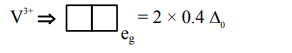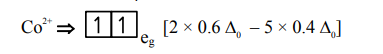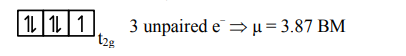Deepak Scored 45->99%ile with Bounce Back Crack Course. You can do it too!

# The Crystal Field Stabilization Energy

Question:

The Crystal Field Stabilization Energy (CFSE) and magnetic moment (spin-only) of an octahedral aqua complex of a metal ion $\left(\mathrm{M}^{2+}\right)$ are $-0.8 \Delta_{0}$ and $3.87 \mathrm{BM}$, respectively. Identify $\left(\mathrm{M}^{2+}\right)$ :

1. $\mathrm{V}^{3+}$

2. $\mathrm{Cr}^{3+}$

3. $\mathrm{Mn}^{4+}$

4. $\mathrm{Co}^{2+}$

Correct Option: , 4

Solution:$=2$ unpaired $\mathrm{e}^{-}$

$\mu=2.89 \mathrm{Bm}$$=-0.8 \Delta_{0}$hence $\mathrm{d}^{7}$ configuration is of $\mathrm{Co}^{2+}$ Ans.